#### 期刊菜单

Seismic Vulnerability Analysis of Steel-Concrete Composite Structures Considering Time-Dependent Damage of Materials
DOI: 10.12677/HJCE.2019.87145, PDF, HTML, XML, 下载: 734  浏览: 1,094  国家自然科学基金支持

Abstract: Aiming at the complex high-rise steel-concrete composite structures and considering the deterio-ration process of concrete materials during service period, a seismic vulnerability analysis method considering the time-varying characteristics of materials is proposed. Based on incremental dynamic analysis (IDA), the dynamic response of the structure subjected to earthquake during different service years is obtained, and the seismic vulnerability curve of the structure is obtained by combining the seismic damage model and theoretical seismic vulnerability analysis method. Taking a typical complex high-rise steel-concrete composite structure as an example, 15 seismic waves are selected and IDA analysis is conducted based on MIDAS GEN software. Then the designed vulnerability curves and time-varying vulnerability curves were drawn to evaluate the seismic performance of the structure during service periods. The results show that considering the time-varying characteristics of materials, the seismic behavior of composite structures can be analyzed and evaluated more accurately. The seismic vulnerability curve shows a certain time-varying characteristic, which is different from the designed curve which does not consider the time-varying performance of the material. In view of the important position of such structures, the change of the seismic vulnerability with the time-varying properties of materials is worth noting. The seismic vulnerability analysis method for composite structures considering time-varying characteristics proposed in this paper can directly reflect the difference in seismic performance of structures during different service periods and provide a basis for predicting and preventing earthquake disaster.

1. 引言

2. 复杂高层型钢–混凝土组合结构时变地震易损性分析

2.1. 混凝土材料时变模型

${f}_{c}\left(t\right)={f}_{cu,k}\left(-2.861×{10}^{-4}{t}^{2}+1.384×{10}^{-2}t+0.8769\right)$ (1)Figure 1. Technical route for seismic vulnerability analysis considering time-varying properties of materials

${E}_{t}=\frac{{10}^{5}}{2.2+\frac{33}{{f}_{c}\left(t\right)}}$ (2)

2.2. 地震损伤模型

$D=\left\{\begin{array}{l}0.1\frac{\Delta u}{\Delta {u}_{1}}\text{\hspace{0.17em}}\text{\hspace{0.17em}}\text{\hspace{0.17em}}\text{\hspace{0.17em}}\text{\hspace{0.17em}}\text{\hspace{0.17em}}\text{\hspace{0.17em}}\text{\hspace{0.17em}}\text{\hspace{0.17em}}\text{\hspace{0.17em}}\text{\hspace{0.17em}}\text{\hspace{0.17em}}\text{\hspace{0.17em}}\text{\hspace{0.17em}}\text{\hspace{0.17em}}\text{\hspace{0.17em}}\text{\hspace{0.17em}}\text{\hspace{0.17em}}\text{\hspace{0.17em}}\text{\hspace{0.17em}}\text{\hspace{0.17em}}\text{\hspace{0.17em}}\text{\hspace{0.17em}}\text{\hspace{0.17em}}\text{\hspace{0.17em}}\Delta u<\Delta {u}_{1}\\ 0.1\frac{\Delta u-\Delta {u}_{1}}{\Delta {u}_{2}-\Delta {u}_{1}}+0.1\begin{array}{cc}& \Delta {u}_{1}\le \Delta u<\Delta {u}_{2}\end{array}\\ 0.3\frac{\Delta u-\Delta {u}_{2}}{\Delta {u}_{3}-\Delta {u}_{2}}+0.2\begin{array}{cc}& \Delta {u}_{2}\le \Delta u<\Delta {u}_{3}\end{array}\\ 0.3\frac{\Delta u-\Delta {u}_{3}}{\Delta {u}_{4}-\Delta {u}_{3}}+0.5\begin{array}{cc}& \Delta {u}_{3}\le \Delta u<\Delta {u}_{4}\end{array}\\ 0.2\frac{\Delta u-\Delta {u}_{4}}{\Delta u}+0.8\text{\hspace{0.17em}}\text{\hspace{0.17em}}\text{\hspace{0.17em}}\text{\hspace{0.17em}}\text{\hspace{0.17em}}\text{\hspace{0.17em}}\text{\hspace{0.17em}}\text{\hspace{0.17em}}\text{\hspace{0.17em}}\text{\hspace{0.17em}}\Delta u>\Delta {u}_{4}\end{array}$ (3)

$\left[\Delta u\right]=\left[\theta \right]h$ (4)Table 1. Classification of seismic performance level of complex high-rise steel-concrete composite structures 

2.3. 结构地震易损性分析

1) 按照地震波的选取原则，选择足够数量的地震波，并对地震波进行预处理，即控制地震持续时间、截取代表性波段以及进行调幅处理。

2) 选取合理的抗震性能指标，定义合理的结构损伤破坏状态以及相应的损伤极限值，建立地震损伤模型。

3) 结合所选的调幅地震波对型钢-混凝土组合结构的有限元模型进行一系列非线性时程分析(IDA)，得到层间位移角和层间位移等地震响应。

4) 基于建立的地震损伤模型，计算与各结构响应值对应的损伤值。

5) 将地震损伤值对数化后进行线性回归分析，得到结构地震损伤对数值与地震波参数(地面加速度峰值(PGA))对数的线性回归方程，如式(5)所示。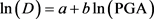(5)

6) 计算结构在不同破坏阶段的超越概率，绘制地震易损性曲线。结构超越目标极限性能的失效概率为：

${P}_{f}=P\left[\frac{{D}_{d}}{{D}_{c}}\ge 1\right]$ (6)

${P}_{f}\left(\text{PGA}\right)=\Phi \left(\frac{\mathrm{ln}\left({D}_{d}/{D}_{c}\right)}{\sqrt{{\beta }_{c}^{2}+{\beta }_{d}^{2}}}\right)$ (7)

3. 算例分析

3.1. 工程模型的建立Table 2. Material and section size list of components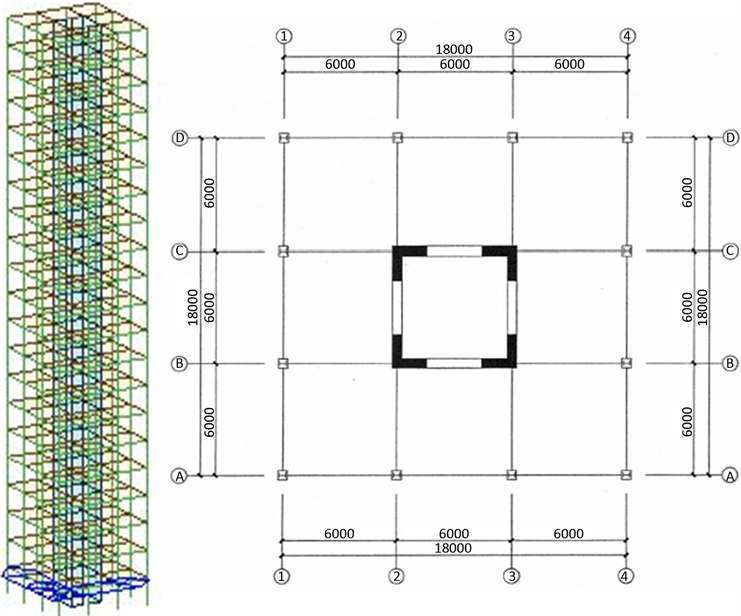Figure 2. Standard floor plan and schematic diagram of structure

${\left\{P\right\}}^{\text{T}}=\left\{{F}_{x1},{M}_{x1},{F}_{y1},{M}_{y1},{F}_{z1},{M}_{z1},{F}_{x2},{M}_{x2},{F}_{y2},{M}_{y2},{F}_{z2},{M}_{z2}\right\}$ (8)

${\left\{\Delta \right\}}^{\text{T}}=\left\{{u}_{1},{\theta }_{x1},{v}_{1},{\theta }_{y1},{\omega }_{1},{\theta }_{z1},{u}_{2},{\theta }_{x2},{v}_{2},{\theta }_{y2},{\omega }_{2},{\theta }_{z2}\right\}$ (9)

3.2. 结构增量动力分析(IDA)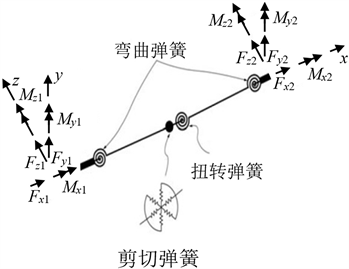(a) 3D梁–柱单元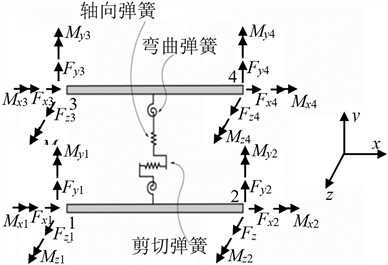(b) 墙单元

Figure 3. Schematic diagram of plastic hinge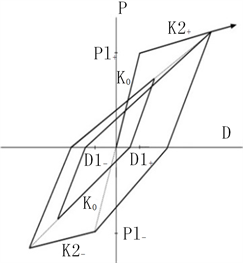(a) 克拉夫双折线模型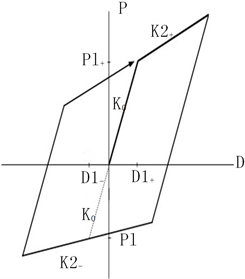(b) 标准双折线模型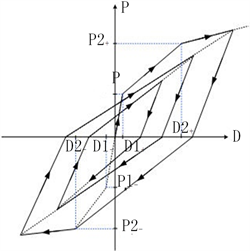(c) 修正武田三折线模型

Figure 4. Hysteresis model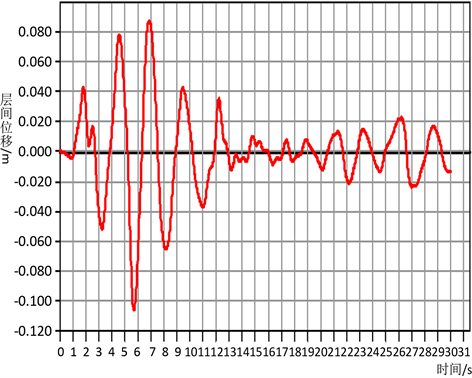Figure 5. Top layer displacement time history diagram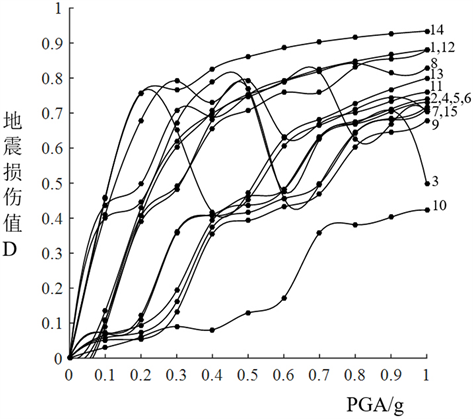Figure 6. IDA curves

Table 3. Selected seismic waves

3.3. 地震易损性分析

3.4. 结构时变地震易损性分析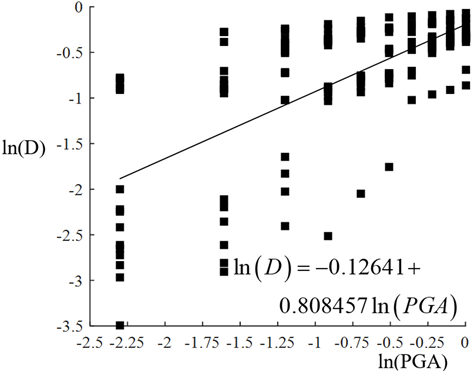Figure 7. Linear regression analysis and equations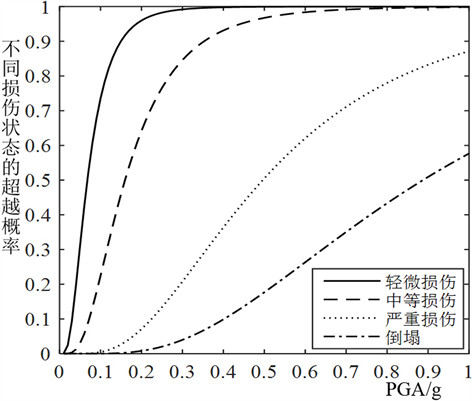Figure 8. Design seismic vulnerability curves

Table 4. Compressive strength and elastic modulus of concrete at different ages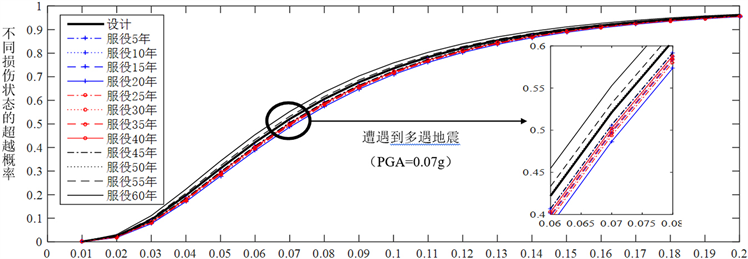Figure 9. Time-varying seismic vulnerability curves for minor damageFigure 10. Time-varying seismic vulnerability curves of moderate damage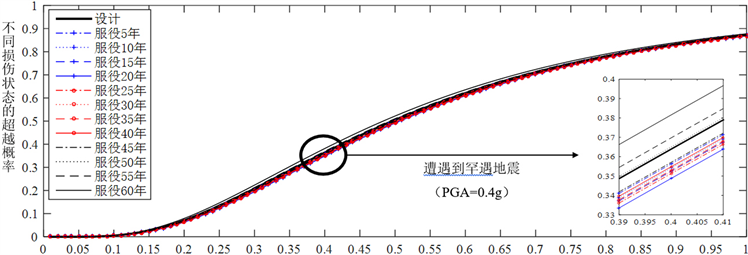Figure 11. Time-varying seismic vulnerability curves of severe damage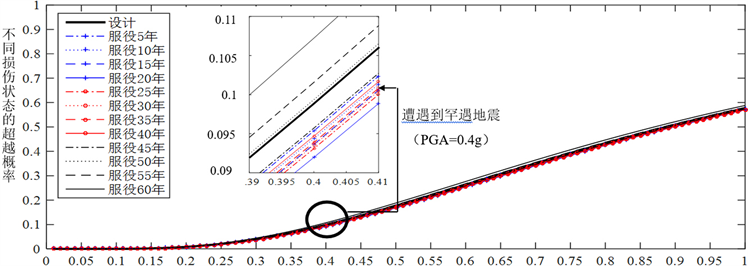Figure 12. Collapse time-varying seismic vulnerability curves

4. 结论

1) 考虑材料的性能劣化和时变特征，可以更为准确地分析和评估不同服役期间的复杂高层型钢–混凝土组合结构的抗震性能；

2) 地震易损性曲线直观地反映了某一水平的地震动作用下组合结构的破坏概率。就本文的组合结构而言，设计地震易损性总体上随地震强度增大而不断提高。当地震动强度较小时，该结构处于轻微和中等损伤状态且损伤发展十分迅速，表明此时结构对地震动强度的变化十分敏感。结构发生严重损伤和倒塌的概率随地震强度增长的速率较为平缓，且结构倒塌的超越概率要远小于前三种损伤，说明该型钢–混凝土结构具有良好的安全性，不易发生倒塌。

3) 地震作用下组合结构的地震易损性曲线呈现出一定的时变特性，与不考虑材料时变性能的设计地震易损性曲线存在差异。在服役的前期超越概率的改变尚不明显，但在服役后期，不同损伤的超越概率已经明显超过了设计概率值，例如，在服役第60年遭遇多遇地震时，发生轻微损伤的概率比设计概率高了约7.8%。在服役第60年遭遇罕遇地震时，发生严重损伤的概率比设计概率高了约5.5%，发生倒塌的概率比设计概率高了约11.3%。这是因为材料弹性模量等性能指标的改变会导致结构刚度随之变化，从而影响了结构的抗震性能。因此，本文提出的基于材料时变特性的钢-混组合结构地震易损性分析方法能够直观地反映结构在不同服役期内抗震性能的差异，可以为结构预测预防地震灾害提供一定的依据。

  高向玲, 颜迎迎, 李杰. 一般大气环境下混凝土经时抗压强度的变化规律[J]. 土木工程学报, 2015, 48(1): 19-26.  王振宇, 刘晶波. 建筑结构地震损伤评估的研究进展[J]. 世界地震工程, 2001, 17(3): 43-48.  沈祖炎, 董宝, 曹文衔. 结构损伤累积分析的研究现状和存在的问题[J]. 同济大学学报(自然科学版), 1997(2): 135-140.  Messina, A., Williams, E.J. and Contursi, T. (1998) Structural Damage Detection by a Sensitive and Statistical-Based Method. Journal of Sound & Vibration, 216, 791-808. https://doi.org/10.1006/jsvi.1998.1728  王立明, 顾祥林, 沈祖炎, 等. 钢筋混凝土结构的损伤累积模型[C]//第六届全国结构工程学术会议论文集: 第二卷. 南宁: 清华大学出版社, 1997: 44-49.  Ang, H.S. (1985) Seismic Damage Analysis of Reinforced Concrete Buildings. Journal of Structural Engineering, 111, 740-757. https://doi.org/10.1061/(ASCE)0733-9445(1985)111:4(740)  鲍烜平. 腐蚀服役环境条件下斜拉桥拉索地震易损性[D]: [硕士学位论文]. 大连: 大连理工大学, 2014.  赵人达, 高能, 贾毅, 等. 基于IDA的大跨连续梁桥地震易损性分析[J]. 沈阳建筑大学学报(自然科学版), 2017(4): 672-679.  Karim, K.R. and Yamazaki, F. (2001) Effect of Earthquake Ground Motions on Fragility Curves of Highway Bridge Piers Based on Numerical Simu-lation. Earthquake Engineering & Structural Dynamics, 30, 1839-1856. https://doi.org/10.1002/eqe.97  Shinozuka, M., Feng, M.Q., Kim, H.K., et al. (2000) Nonlinear Static Proce-dure for Fragility Curve Development. Journal of Engineering Mechanics, 126, 1287-1295. https://doi.org/10.1061/(ASCE)0733-9399(2000)126:12(1287)  庞于涛, 袁万城, 党新志, 等. 考虑材料劣变过程的桥梁地震易损性分析[J]. 同济大学学报(自然科学版), 2013, 41(3): 348-354.  Hwang, H., 刘晶波. 地震作用下钢筋混凝土桥梁结构易损性分析[J]. 土木工程学报, 2004, 37(6): 47-51.  何益斌, 李艳, 沈蒲生. 基于性能的高层混合结构地震易损性分析[J]. 工程力学, 2013, 30(8): 142-147, 162.  王若竹. 复杂高层钢–混凝土结构地震损伤时变特性研究[D]: [硕士学位论文]. 南京: 东南大学, 2016.  王莹, 李兆霞, 王若竹. 复杂高层钢–混凝土结构地震损伤时变特性研究[J]. 湖南大学学报(自然科学版), 2017, 44(9): 52-62, 127.